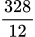Price Comparison between Appsero and Other Platforms
Share
Explore

#Appsero+Paddle vs WooCommerce+Stripe

Calculation Table - Appsero & Paddle
18
Search
Product Price
Appsero Fee
With Appsero 50% Discount 🥳
1
50
$25.00$20.00
25
$62.50$87.50
$107.50$97.50
2
250
$25.00$20.00
125
$312.50$437.50
$457.50$447.50
3
500
$25.00$20.00
250
$625.00$875.00
$895.00$885.00
4
1000
$25.00$57.50
500
$1,250.00$1,750.00
$1,807.50$1,778.75
5
1500
$25.00$57.50
750
$1,875.00$2,625.00
$2,682.50$2,653.75
6
2500
$25.00$82.50
1250
$3,125.00$4,375.00
$4,457.50$4,416.25
7
3500
$25.00$82.50
1750
$4,375.00$6,125.00
$6,207.50$6,166.25
8
5000
$25.00$157.50
2500
$6,250.00$8,750.00
$8,907.50$8,828.75
9
7500
$25.00$157.50
3750
$9,375.00$13,125.00
$13,282.50$13,203.75
10
10000
$25.00$290.83
5000
$12,500.00$17,500.00
$17,790.83$17,645.42
There are no rows in this table
💰 Cost for WooCommerce (Using WooCommerce Subscription, Software Add-on & Stripe Payment Gateway)
WooCommerce Plugins Cost
1
Search
Plugin Name
Annual Cost
1
$129.00 2 WooCommerce Subscriptions$199.00
There are no rows in this table
$328.00 Sum Monthly Cost WooCommerce Fee=$27.33
Cost for WooCommerce with Plugins
18
Search
Product Price
WooCommerce Fee
Stripe 3.4%
Stripe 50C
Total Cost with WooCommerce + Stripe
1
50
$25.00$27.33
$42.50 25$94.83
2
250
$25.00$27.33
$212.50 125$364.83
3
500
$25.00$27.33
$425.00 250$702.33
4
1000
$25.00$27.33
$850.00 500$1,377.33
5
1500
$25.00$27.33
$1,275.00 750$2,052.33
6
2500
$25.00$27.33
$2,125.00 1250$3,402.33
7
3500
$25.00$27.33
$2,975.00 1750$4,752.33
8
5000
$25.00$27.33
$4,250.00 2500$6,777.33
9
7500
$25.00$27.33
$6,375.00 3750$10,152.33
10
10000
$25.00$27.33
$8,500.00 5000$13,527.33
There are no rows in this table
💰 Appsero 🆚 WooCommerce Price Comparison
Price Comparison: Appsero + Paddle vs WooCommerce + Stripe
18
Search
Product Price
Total Cost with WooCommerce + Stripe
1
50
$25.00$107.50
$94.83 2 250$25.00
$457.50$364.83
3
500
$25.00$895.00
$702.33 4 1000$25.00
$1,807.50$1,377.33
5
1500
$25.00$2,682.50
$2,052.33 6 2500$25.00
$4,457.50$3,402.33
7
3500
$25.00$6,207.50
$4,752.33 8 5000$25.00
$8,907.50$6,777.33
9
7500
$25.00$13,282.50
$10,152.33 10 10000$25.00
$17,790.83$13,527.33
There are no rows in this table
💰 Total Cost Percentage Comparing Revenue
Total Cost Percentage When You Use WooCommerce+Stripe 🆚 Appsero+Paddle 2
18
Search
Product Price
Total Revenue
% Cost Appsero
Percent Cost WooCommerce with Stripe
Difference
1
50
$25.00$1,250.00
8.6%
7.59%
1.01%
2
250
$25.00$6,250.00
7.32%
5.84%
1.48%
3
500
$25.00$12,500.00
7.16%
5.62%
1.54%
4
1000
$25.00$25,000.00
7.23%
5.51%
1.72%
5
1500
$25.00$37,500.00
7.15%
5.47%
1.68%
6
2500
$25.00$62,500.00
7.13%
5.44%
1.69%
7
3500
$25.00$87,500.00
7.09%
5.43%
1.66%
8
5000
$25.00$125,000.00
7.13%
5.42%
1.71%
9
7500
$25.00$187,500.00
7.08%
5.41%
1.67%
10
10000
$25.00$250,000.00
7.12%
5.41%
1.71%
There are no rows in this table
Graph Comparison
How much you spend in each of the platforms
18